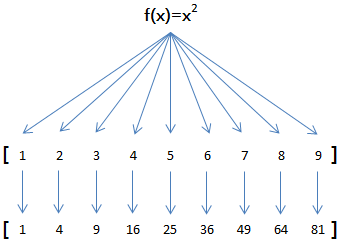# map/reduce

Python内建了`map()``reduce()`函数。``````>>> def f(x):
...     return x * x
...
>>> map(f, [1, 2, 3, 4, 5, 6, 7, 8, 9])
[1, 4, 9, 16, 25, 36, 49, 64, 81]
``````

`map()`传入的第一个参数是`f`，即函数对象本身。

``````L = []
for n in [1, 2, 3, 4, 5, 6, 7, 8, 9]:
L.append(f(n))
print L
``````

``````>>> map(str, [1, 2, 3, 4, 5, 6, 7, 8, 9])
['1', '2', '3', '4', '5', '6', '7', '8', '9']
``````

``````reduce(f, [x1, x2, x3, x4]) = f(f(f(x1, x2), x3), x4)
``````

``````>>> def add(x, y):
...     return x + y
...
>>> reduce(add, [1, 3, 5, 7, 9])
25
``````

``````>>> def fn(x, y):
...     return x * 10 + y
...
>>> reduce(fn, [1, 3, 5, 7, 9])
13579
``````

``````>>> def fn(x, y):
...     return x * 10 + y
...
>>> def char2num(s):
...     return {'0': 0, '1': 1, '2': 2, '3': 3, '4': 4, '5': 5, '6': 6, '7': 7, '8': 8, '9': 9}[s]
...
>>> reduce(fn, map(char2num, '13579'))
13579
``````

``````def str2int(s):
def fn(x, y):
return x * 10 + y
def char2num(s):
return {'0': 0, '1': 1, '2': 2, '3': 3, '4': 4, '5': 5, '6': 6, '7': 7, '8': 8, '9': 9}[s]
return reduce(fn, map(char2num, s))
``````

``````def char2num(s):
return {'0': 0, '1': 1, '2': 2, '3': 3, '4': 4, '5': 5, '6': 6, '7': 7, '8': 8, '9': 9}[s]

def str2int(s):
return reduce(lambda x,y: x*10+y, map(char2num, s))
``````

lambda函数的用法在后面介绍。

### 练习

Python提供的`sum()`函数可以接受一个list并求和，请编写一个`prod()`函数，可以接受一个list并利用`reduce()`求积。